﻿ 一种漏电流检测快速保护方法研究

# 一种漏电流检测快速保护方法研究 Study on Leakage Current Detection and Fast Protection Method

Abstract: In this paper, a leakage current detection and protection method based on oscillating circuit and differential circuit is proposed for the safety requirements of non-isolated power electronic devices connected to power grid. The leakage current protection circuit consists of transformer, self-excited oscillator, second-order active low-pass filter circuit, differential detection circuit and existing control chips in the system. The leakage current signal detected by the leakage current detection circuit is sampled through the digital control chip in the device, and the leakage current value sampled is compared with the specified value in the safety standard, thus forming a hierarchical protection. For the excessive leakage current, the differential circuit and software in the leakage current detection circuit studied can achieve rapid protection. Finally, the validity of this leakage current detection and protection method is verified by experiments in three-phase bidirectional PWM rectifier applied to the front end of motor driver.

1. 引言

2. 漏电流检测和保护方法

2.1. 漏电流检测电路工作原理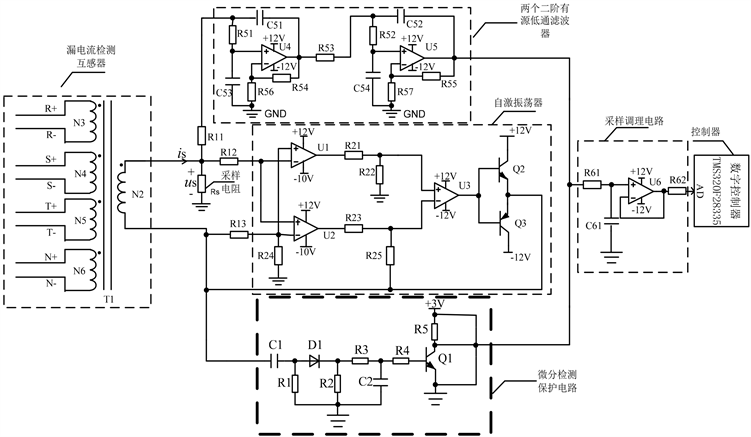Figure 1. General schematic diagram of leakage current detection and protection circuit

1) 自激振荡电路工作原理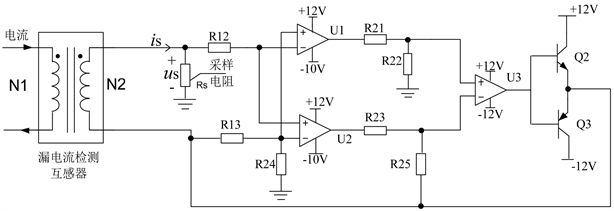Figure 2. Self-excited oscillation circuit

2) 二阶有源低通滤波电路工作原理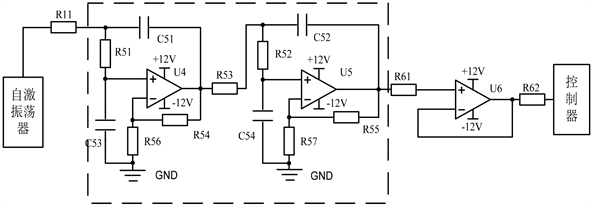Figure 3. Second-order active low-pass filter circuit

$A\left(s\right)=\frac{{V}_{o}\left(s\right)}{{V}_{i}\left(s\right)}=\frac{{A}_{VF}}{1+\left(3-{A}_{VF}\right)sCR+{\left(sCR\right)}^{2}}$ (1)

${A}_{VF1}=1+\frac{{\text{R}}_{54}}{{\text{R}}_{56}}$ (2)

${f}_{c1}=\frac{1}{2\text{π}\sqrt{\text{C}51\cdot \text{C}53\cdot \text{R}11\cdot \text{R}51}}$ (3)

3) 微分检测保护电路的工作原理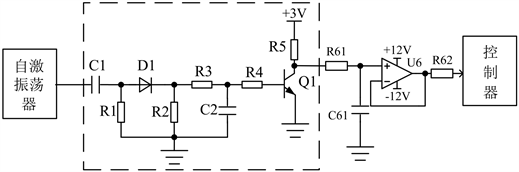Figure 4. Differential detection protection circuit

2.2. 漏电流检测保护原理

1) 漏电流较小时的响应

2) 漏电流较大时的响应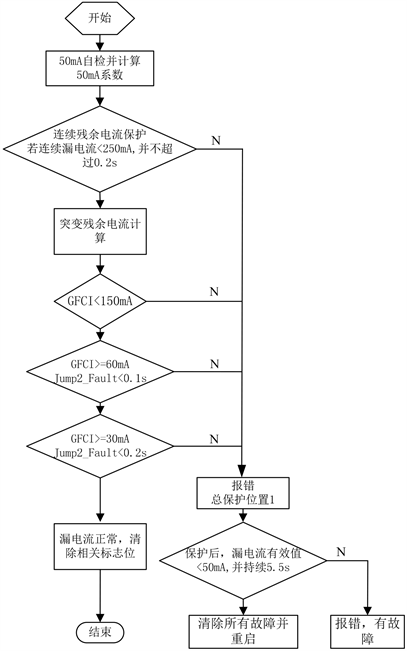Figure 5. Flow chart of leakage current detection and protection program

3. 漏电流检测保护电路的设计

3.1. 自激振荡器的设计

1) 磁芯选取

2) 计算原副边绕组匝数

${A}_{e}=\left(\text{OD-ID}\right)\cdot \text{HD}=20\text{\hspace{0.17em}}{\text{mm}}^{2}$ (4)

$Q=\frac{\text{π}\cdot {\text{ID}}^{2}}{4}=176.7\text{\hspace{0.17em}}{\text{mm}}^{2}$ (5)

${N}_{1}=\frac{{V}_{c}\cdot {T}_{s}}{4{B}_{m}\cdot {A}_{e}}=73.171$ (6)

3) 线径选取

${I}_{N1}=\frac{{I}_{N3}}{{N}_{1}}\cdot {N}_{3}=4.11\text{\hspace{0.17em}}\text{mA}$ (7)

$nn1=\frac{{I}_{N1}}{J\cdot {S}_{d3}}=0.058\text{\hspace{0.17em}}{\text{m}}^{-2}\cdot {\text{kg}}^{-1}\cdot {\text{s}}^{2}$ (8)

$nn2=\frac{50\cdot {10}^{-3}}{J\cdot {S}_{d3}}=0.707\text{\hspace{0.17em}}{\text{m}}^{-2}\cdot {\text{kg}}^{-1}\cdot {\text{s}}^{2}$ (9)

$nn3=\frac{25}{J×{S}_{d4}}=6.25\text{\hspace{0.17em}}{\text{m}}^{-2}\cdot {\text{kg}}^{-1}\cdot {\text{s}}^{2}$ (10)

$k=\frac{{N}_{1}\cdot n{n}_{1}\cdot {S}_{d3}+{N}_{2}\cdot n{n}_{2}\cdot {S}_{d3}+4{N}_{3}\cdot n{n}_{3}\cdot {S}_{d4}}{Q}=0.078$ (11)

3.2. 二阶有源低通滤波器的设计

${A}_{VF1}=1+\frac{\text{R}54}{\text{R56}}=2$ (12)

${f}_{c1}=\frac{1}{2\text{π}\sqrt{\text{C51}\cdot \text{C53}\cdot \text{R11}\cdot \text{R51}}}=1.071\text{\hspace{0.17em}}\text{kHz}$ (13)

${A}_{VF2}=1+\frac{\text{R55}}{\text{R57}}=2.538$ (14)

${f}_{c2}=\frac{1}{2\text{π}\sqrt{\text{C52}\cdot \text{C54}\cdot \text{R}52\cdot \text{R53}}}=1.329\text{\hspace{0.17em}}\text{kHz}$ (15)

${A}_{VF}={A}_{VF1}\cdot {A}_{VF2}=5.077$ (16)

3.3. 漏电流检测微分保护电路参数设计

$\left\{\begin{array}{c}{u}_{c}{}_{\left(80\text{\hspace{0.17em}}\text{μs}\right)}=12\cdot \left(1-{e}^{-\frac{80\cdot {10}^{-6}}{{\tau }_{1}}}\right)\ge 0.6\text{\hspace{0.17em}}\text{V}\\ {u}_{c}{}_{\left(80\text{\hspace{0.17em}}\text{μs}\right)}\ge 3.3\text{\hspace{0.17em}}\text{V}\end{array}$ (17)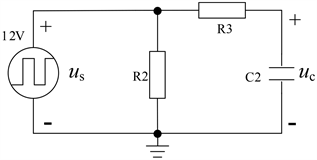Figure 6. RC circuits

${\tau }_{1}={\text{R}}_{3}\cdot {\text{C}}_{2}$ (18)

${\tau }_{1}={\text{R}}_{3}\cdot {\text{C}}_{2}\le 2.5\cdot {10}^{-4}$ (19)

${u}_{c}{}_{\left(160\text{\hspace{0.17em}}\text{μs}\right)}={u}_{c}{}_{\left(80\text{\hspace{0.17em}}\text{μs}\right)}{e}^{-\frac{80\cdot {10}^{-6}}{{\tau }_{2}}}\ge 3.3\text{\hspace{0.17em}}\text{V}$ (20)

${\tau }_{2}=\left({\text{R}}_{3}+{\text{R}}_{2}\right)\cdot {\text{C}}_{2}$ (21)

${\tau }_{2}=\left({\text{R}}_{3}+{\text{R}}_{2}\right)\cdot {\text{C}}_{2}\ge 1.16\cdot {10}^{-4}$ (23)

4. 实验结果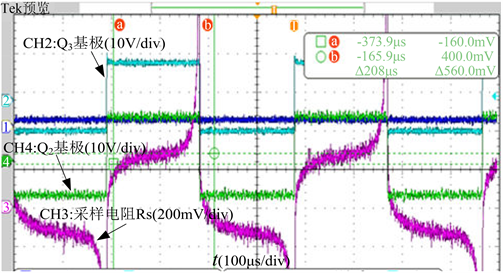Figure 7. Self-excited oscillation waveform with small leakage current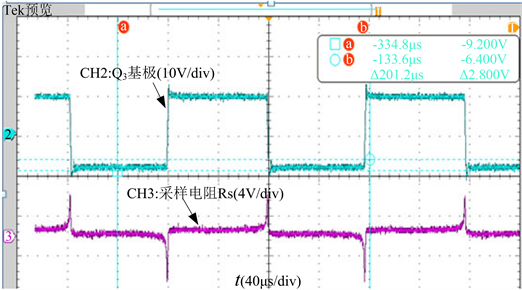Figure 8. Self-excited oscillation waveform with large leakage current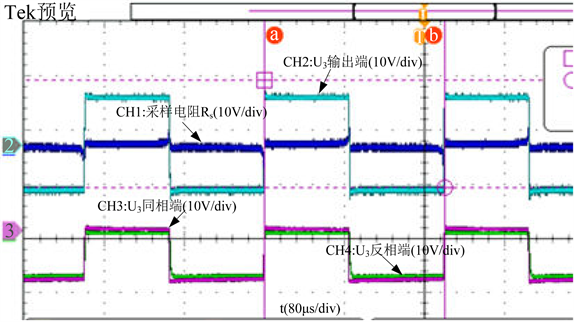Figure 9. Self-excited oscillation waveform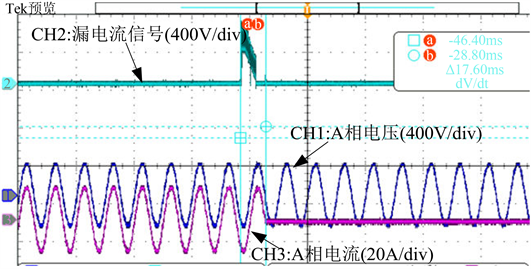Figure 10. Leakage current detection protection waveform

5. 结论

 刘建科, 韩晨, 于克锐, 陈永佳, 崔永宏, 张诚. 基于SVPWM光伏并网逆变器漏电流抑制研究[J]. 可再生能源, 2016, 34(9): 1287-1292.

 Lu, Q.Y., et al. (2017) Method for On-Line Detection of Leakage Current of Power Supply SPD. Chinese Institute of Electronics IEEE Beijing Section: IEEE Beijing Section, Beijing, 28-31 October 2017, 180-190.
https://doi.org/10.1109/EMC-B.2017.8260467

 Gunsal, I., Stone, D.A. and Foster, M.P. (2018) A Unique Pulse Width Modulation to Reduce Leakage Current for Cascaded H-Bridge Inverters in PV and Battery Energy Storage Applications. Energy Procedia, 151, 12-17.
https://doi.org/10.1016/j.egypro.2018.09.032

 王付胜, 于世能. 级联H桥逆变器漏电流分析与抑制[J]. 电工技术学报, 2017, 32(S2): 103-110.

 张志文, 周滔, 刘军, 范威. 三相无变压器型H7光伏逆变器漏电流分析抑制[J]. 电源学报, 2018, 16(4): 107-112.

 贲冰. 三电平四桥臂光伏逆变器漏电流抑制研究[J]. 中国电机工程学报, 2018, 38(14): 4194-4201.

 郭小强, 朱铁影. 新型非隔离型三相三电平光伏并网逆变器及其漏电流抑制研究[J]. 电工技术学报, 2018, 33(1): 26-37.

 童树卫, 余志勇, 钱彬. 一种用于非隔离光伏并网逆变器漏电流检测的电路设计与实现[J]. 电子科学技术, 2015, 2(2): 162-167.

 Magtron Marketing. 漏电流检测基本原理以及在电动汽车充电桩中漏电流保护方法的选择[J]. 世界电子元器件, 2018(7): 32-37.

Top